### Create an Account

Home / Questions / To show that the Debye specific heat capacity a t low tempera1ure can be determined from m...

# To show that the Debye specific heat capacity a t low tempera1ure can be determined from measurements of the velocity of sound show that Where and show that the specific heat per

To show that the Debye specific heat capacity a t low tempera1ure can be determined from measurements of the velocity of sound, (a) show that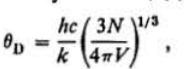Where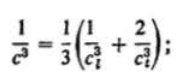and (b) show that the specific heat per kilogram cv is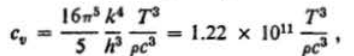where p is the density of the material. (c) Calculate the average value of the sound velocity in copper. For copper, p is approximately 9000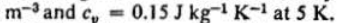(d) Calculate a value for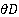and for vm for copper. (e) Calculate the value of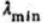and compare to the interatomic spacing, assuming that copper has a cubic structure.

May 08 2020 View more View LessSubscribe To Get Solution